# Catch up on the latest AI articles# What Is Self-Regulation That Addresses The Main Failures Of Semantic Segmentation?

3 main points
✔️ Addresses key failures in semantic segmentation
✔️ Proposes a Self-Regulation loss that can be applied to various backbones
✔️ Improves performance of existing methods on weakly-supervised/supervised segmentation tasks

written by Zhang DongZhang HanwangTang JinhuiHua XianshengSun Qianru
(Submitted on 22 Aug 2021)

Subjects: Computer Vision and Pattern Recognition (cs.CV)code：The images used in this article are from the paper, the introductory slides, or were created based on them.

## first of all

Research in semantic segmentation, which predicts the class label corresponding to each pixel in an image, has made significant progress due to advances such as deep CNNs. For example, EfficientFCN achieves an mIOU of about 85% on PASCAL Context, a complex natural image segmentation task.

In the paper presented in this article, we proposed a loss called Self-Regulation to address this main failure in existing semantic segmentation models.

## On the main failures in semantic segmentation

The original paper cites two major examples of failures in semantic segmentation.

### Failure Example 1: Missing a small object or a small part of an object

The first failure is illustrated for example in the following figure.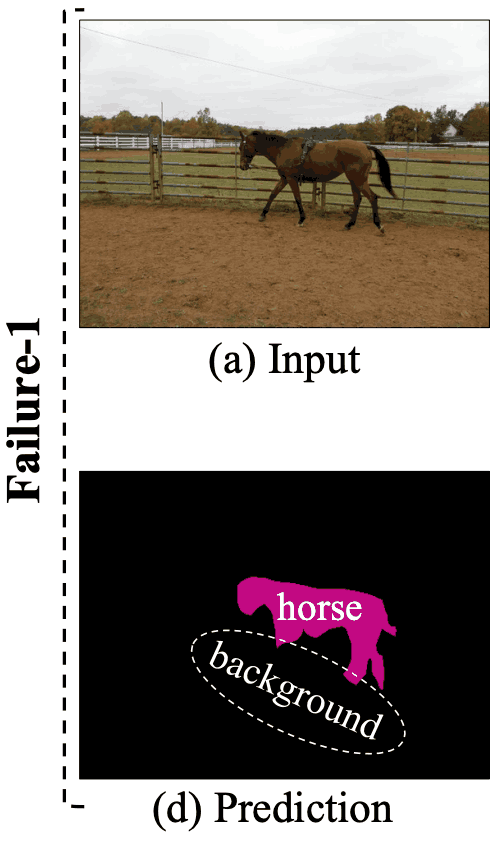This figure shows the prediction result by DeepLab-v2. As you can see in the figure, the prediction of the horse's torso is successful, but the prediction of the leg part is not.

### Failure Example 2: Doing another class label prediction on a part of a larger object

The second case of failure is as follows.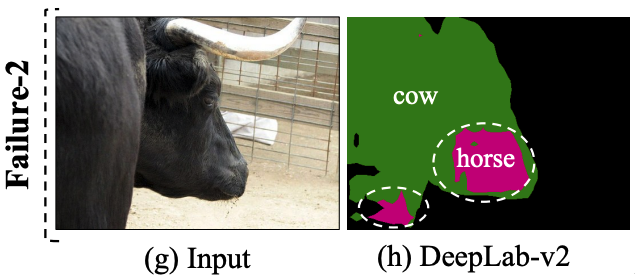This figure incorrectly predicts some of the cows, which make up about half of the image, like horses.

Self-Regulation, the loss function proposed in the original paper, aims to address these two main failures.

### Dealing with Failure Example 1

The following figure visualizes the features of shallow and deep layers during semantic segmentation prediction for horse images in DeepLab-v2.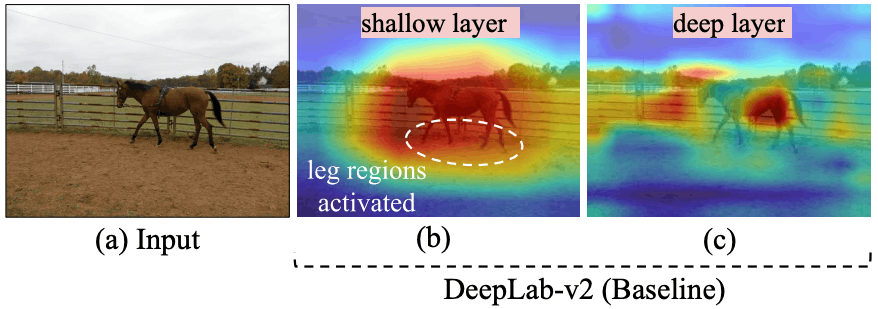This figure shows that at the shallow layer, the horse's (unsuccessfully predicted) legs are also well activated, while at the deep layer, the horse is influenced by the background and the leg information is neglected.

The proposed method effectively transfers the shallow layer information to the deep layer by introducing a distillation loss where the shallow layer feature map is the teacher and the deep layer feature map is the student.

### Dealing with Failure Example 2

In the aforementioned failure example 2, a part of a cow was wrongly predicted as a horse. To deal with such cases, the proposed method uses image-level classification loss.

For example, if the class prediction for the whole image is "cow", then it is intuitively unnatural to have a class for "horse" in the image.

In the proposed method, as a countermeasure to failure case 2, we introduce a distillation loss where the deep layer classification logit is the teacher and the shallow layer classification logit is the student, in addition to using the existing method, Multi-Exit Architecture loss (MEA loss).

These measures are illustrated in the following figure.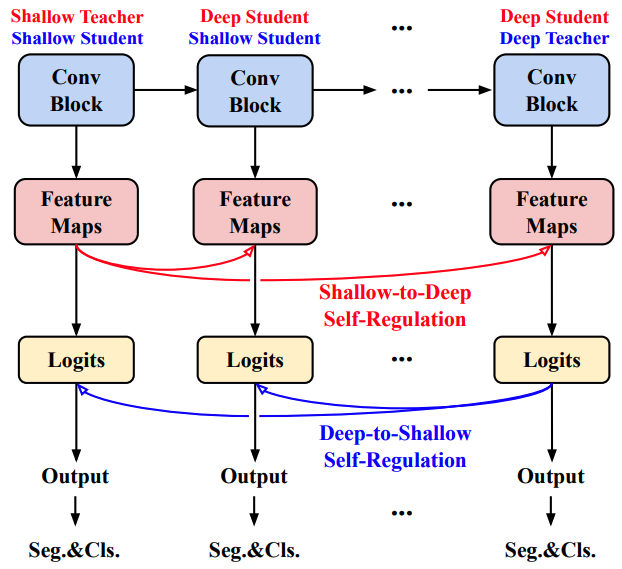As shown in the figure, the shallow layers adjust the deep layers (by distillation loss) in the feature map for each layer, and the deep layers adjust the shallow layers in the classification logit, thus addressing the aforementioned issues.

## [Proposed method (Self-Regulation)

Self-Regulation is designed to combine three types of losses. Let's look at them in order.

### Loss 1: Self-Regulation Loss using Feature Map (SR-F)

SR-F is a loss aimed at addressing the aforementioned failure case 1 and aims to encourage feature map information in the shallow layers to be retained in the deep layers. This corresponds to the Shallo-to-Deep Self-Regulation in the preceding figure.

Specifically, for the feature map $T^{}_{\theta}$ of the shallowest layer (Shallow Teacher) and the feature map $S^{}_{\phi}$ of the $i$th layer (Deep Student), the SR-F loss $L_{SR-F}$ is obtained as follows

$L_{ce}(T^{}_{\theta},S^{}_{\phi})=-\frac{1}{M}\sum^M_{j=1} \sigma(t_j)log(\sigma(s_j))$

$L^{\tau}_{ce}(T^{}_{\theta},S^{}_{\phi})=-\tau^2 \frac{1}{M}\sum^M_{j=1} \sigma(t_j)^{1/\tau}log(\sigma(s_j)^{1/\tau})$

$L_{SR-F}=\sum^N_{i=2} L^{\tau}_{ce}(T^{}_{\theta},S^{}_{\phi})$

For $L_{ce}$, $t_j$ is the vector corresponding to the $j$th pixel of the feature map $T^{}_{\theta}$, $s_j$ is the vector corresponding to the $j$th pixel of the feature map $S^{}_{\phi}$, $\sigma$ is the per-channel softmax normalization, and $M$ is the width × height of the feature map.

The SR-F loss utilizes temperature scaling, which is commonly used in knowledge distillation, where $\tau$ is a temperature parameter.

### Loss 2: Self-Regulation Loss (SR-L) using Classification Logit

SR-L is a loss aimed at addressing the aforementioned failure case 2, by encouraging the shallow layer to capture the classification logit information in the deep layer, thus increasing the robustness to background noise. This corresponds to Deep-to-Shallow Self-Regulation in the previous figure.

Specifically, for the deepest layer (Deep Teacher) classification logit $T^{[N]}_{\theta}$ and the shallow layer (Shallow Student) feature map $\{S^{[k]}_{\phi}\}^{N-1}\}^{N-1}_{k=1}$, the SR-L loss $L _{SR-F}$ can be obtained as follows.

$L_{SR-L}=\sum^{N-1}_{k=1} L^{\tau}_{ce}(T^{[N]}_{\theta},S^{[k]}_{\phi})$

In addition to these two Self-Regulation losses, we also use the MEA loss (which is not the subject of the paper and will not be discussed in detail). Overall, the loss function is as follows.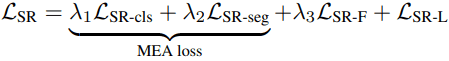$\lambda_1,\lambda_2,\lambda_3$ will be hyperparameters that indicate the weight of each loss.

## experimental results

In our experiments, we evaluate Self-Regulation on two tasks: supervised semantic segmentation (FSSS) and weakly supervised semantic segmentation (WSSS).

WSSS will use two benchmarks, PASCAL VOC 2012 (PC) and MS-COCO 2014 (MC).

FSSS uses two benchmarks, Cityscapes (CS) and PASCAL Context (PAC).

### Ablation studies for each loss

The results of the ablation experiments for the three types of losses (SR-F, SR-L, and MEA) included in the proposed method are as follows.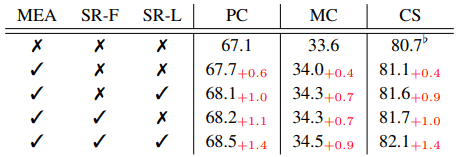It can be seen that the introduction of SR losses has consistently improved the performance.

### Effect of the proposed method on WSSS/FSSS

The results of introducing the proposed method into the baseline model in WSSS are shown in the following table.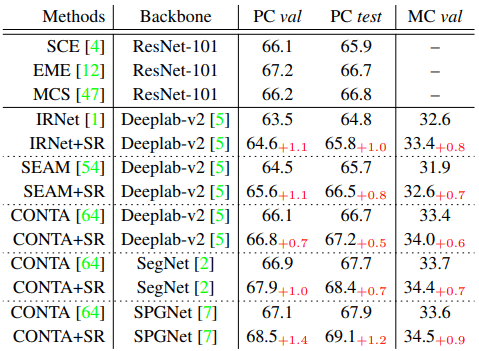The FSSS is as follows.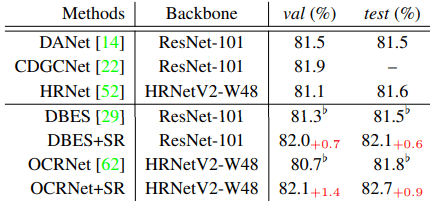The numbers shown in the table represent mIOU (%).

For both WSSS/FSSS tasks, introducing the proposed method into the baseline model (baseline name + SR) consistently improved the performance.

### Computational Cost of the Proposed Method

An example of the increase in the computational cost of introducing the proposed method, SR, into an existing semantic segmentation model is shown below.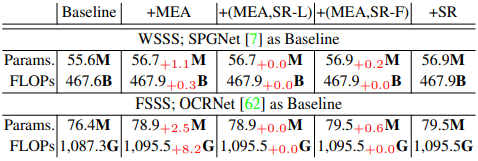For both WSSS and FSSS, the increase in computational cost (number of model parameters and FLOPs) due to the introduction of the proposed method is found to be very small.

## summary

In this article, we introduced Self-Regulation, a loss that aims to address the two main types of failures in semantic segmentation. Self-Regulation effectively adjusts the feature map classification logit of shallow and deep layers by distillation loss from shallow to deep or deep to shallow layers. The proposed method was shown to consistently improve the performance of both weakly supervised and supervised semantic segmentation models.

It is an excellent method in terms of both versatility and efficiency, as it has a low computational cost and can be implemented in various existing models.

If you have any suggestions for improvement of the content of the article,